# Primitive Data Types in C++

## Primitive Data Types in C++ with Examples:

In this article, I am going to discuss Primitive Data Types in C++ with Examples. Please read our previous article where we discussed Why do we need Data Types in C++. At the end of this article, you will learn everything about C++ Primitive Data Types with Examples.

##### Primitive Data Types in C++:

Let us learn about the data types available in the C++ language. Already in the previous article, I have given the idea of why we need data types. Without data type, there is no use in writing the programs. So let us learn what are the data types. Here, we will also learn, how to use them by declaring the variables. So let us overview all the data types available in C++.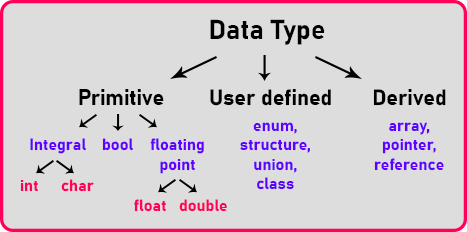We have a chart here that is showing the classification of C++ data types, they are categorized into 3 types. In this article, we will focus on the ‘primitive’ portion. This portion of data types is called primitive data types. Primitive means which are live inside C++ and are directly provided by the compiler. So primitive data types are basic data types of C++.

The primitive data types are of three types in categorization.

1. Integral type, which means there is no decimal point.
2. Boolean means true or false next,
3. Floating-point with the decimal point.

So, this is the broader categorization, under integral we have int and char.

##### Character is integral?

What does it mean? integer and character come under integral means that they will not have a decimal point. Then floating-point and in floating-point, we have two data types i.e. float and double. They are similar only just some differences are there; we will learn about it. So before going into details of this let us have a look at user-defined and derived also.

##### User-Defined Data Types in C++

In User-defined data types, the First one is an enum that is an enumeration. Structure and union. And classes. That’s what C++ is for, C++ supports object orientation. So actual C++ concepts and the programming starts from classes. We will learn a lot of things about it in our upcoming articles.

##### Derived Data Types in C++

Inside derived section, arrays, pointers, and references. You will learn these data types in our upcoming articles.

##### What are the data types?

For data types, we have to know a few things. Let us look at the table.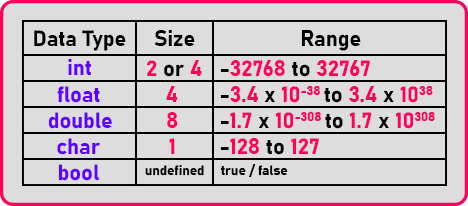The first column represents the Data types and the data types are int, float, double, character, and Boolean. The 2nd column represents their sizes. Size means how many bytes of memory they take.

We can store a small size number. If you want to store a bigger number then what. So that’s what depends on the number that you can store. That’s why every data type has some number of bytes.

The 3rd column of the table shows the range of values that any data type can store. So, their values are also given and you can note these values. You must remember the range of int and char. Then you should remember always next about float and double if you can remember it’s good otherwise it’s not a problem.

##### Integer Data Type in C++

Now let us talk about integer data type in C++. An integer may take either 2 bytes or 4 bytes depending on the version of the compiler you are using. Most of the compilers take 4 bytes only if you are using turbo c, if you know about it then you know it takes 2 bytes, because it opens in the DOS box it goes into DOS shell and run there, so there it takes 2 bytes otherwise integer takes 4 bytes.

But in our discussion every time we will be saying int 2 bytes because when we have to show some calculation so 2 bytes will be easy for us for explanation otherwise taking a bigger size data will be difficult for the explanation.

So, we will assume int takes 2 bytes every time. Now let us understand how this int is getting this range. It is taking 2 bytes so 2 bytes means total how many bits? 16 bits. For better understanding, please have a look at the below image.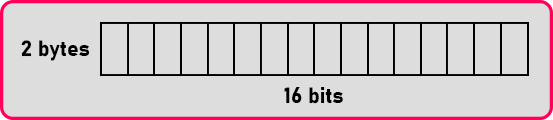So here we have 2 bytes or 16 bits. 16 bits are there, 2 bytes together work as a single variable in their data that is an integer.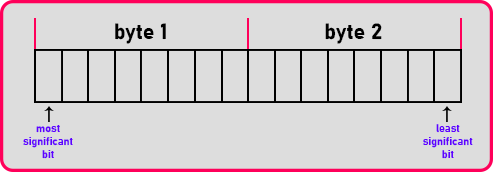The 1st bit is the most significant bit and the last bit is the least significant bit. The 1st bit is reserved for the sign, if this is 1 then it means the number is negative. If that is 0 then it means the number is positive. Then how many bits are remaining for writing a number. Only 15 bits from 0 to 14.

So that’s why the number is stored only in 15 bits.1st bit has to store a sign. Also, that is the reason one bit is reserved. The remaining bits are used for storing a number. So, this is common for all the languages not just C++ it is true for C also and this even is true for Java also.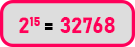This is the largest number that we can store in a 2-byte memory location. Now we will write down the range as 0 to 32767. If I take the range of this number, it will not be starting from 1. It is starting from 0 so it is 0 to 32767. But this is for positive. What about the negative? For negative range will be -32768 to 0. Negative zero is not a number. So that’s why that negative zero is taken as -32768. So, the range is -32768 to 32767.

##### Character Data type in C++:

Character is taking numbers from -128 to 127 and it is taking one bite.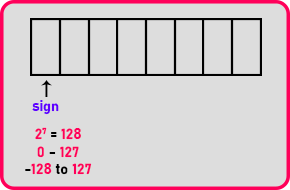One bit is of that sign bit, now how many limits are there 0 to 6 total 7 bits are there i.e. 27 = 128, means 0 to 127 for positive and -128 to 0 for negative.

##### How come a character is a number?

The computer works on the binary number system that is 0 and 1, so they understand only 0 and 1, nothing else. Then how to represent the character. The character should also be represented in 0 and 1. We understand them as the decimal number system. So, character codes are given. So let us see what are codes.

The codes are called ASCII codes American standard code for information interchange. So, for every alphabet or every letter in the English language as well as the special symbols, there is a code given.

##### ASCII Codes:

For a better understanding of ASCII codes, please have a look at the below image.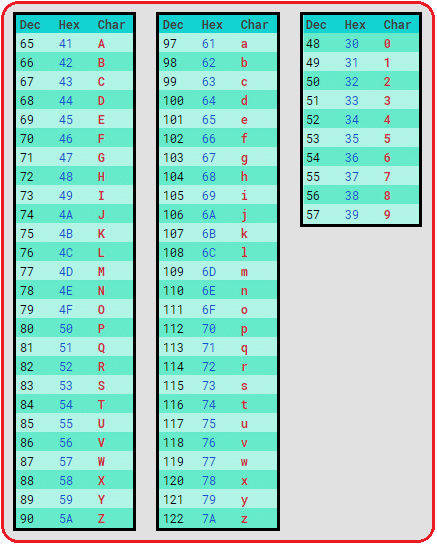Capital A is represented as 65, then B is represented as 66. So, a number is used for representing the character. 65 means it is A. So only on the screen, the printing will be done as A but inside the memory, it is 65.

It is in binary form that all the codes are used for representing characters. 0 is also a symbol or character but we treat it as a number and used them as numbers. But everything on the keyboard is a character so ‘0’ is also a character so for that code is 48 and for 1 is 49 and goes on the last digit is 9 and for 9 is 57.

##### Modifiers in C++

Now let us discuss modifiers. There are two modifiers, using these modifiers we can modify the data types as per our requirement. So quickly let us look at this. There are two identifiers. One is unsigned. And another one is long.

So, we can say unsigned int. Now this integer takes both negative as well as positive. So, what is the range? The range will be 0 to 65535. This means the signed bit is not there all 16 bits are used for storing a number integer become unsigned. So, if you don’t have any negative numbers and you want to get a bigger range you have a larger value then we can go for this. Then you can also see unsigned char. So, when you say unsigned character, the range will be 0 to 255.

Instead of storing alphabets, we can store a number also in a character. So unsigned can be used only with integer and character you cannot use it with any other.

The next one is long. Long we can use it with the integer. So, if int is taking 2 bytes then long int will take 3 bytes and if suppose int is taking 4 bytes then long int will take 8 bytes depending on the compiler. The long can also be taken with double long, it will take 10 bytes.

If you still want a larger value then you can take long double, long float is no meaning because long float itself is double. So, it will not be 16 bytes, it will be 8 bytes.

So that’s all about modifiers, you can use them with limited data types you cannot use unsigned with everything and you can’t use along with everything you cannot have long char. So that’s all about the data types.

##### C++ Primitive Data Types:

These are the Basic data types provided or available in C++. We can call it built-in data types. Let’s categorize further.

• Integer data type: int, short, long
• Floating point data type: Float, double
• Boolean data type: bool
• character data type: char

Each data type occupies a different size in memory. Instead of remembering how much byte int, char, float occupies let’s write a program to identify the size of each data type.

```#include <iostream>
using namespace std;
int main ()
{
cout << "size of int data type:\t" << sizeof (int) << endl;
cout << "size of long data type:\t" << sizeof (long) << endl;
cout << "size of char data type:\t" << sizeof (char) << endl;
cout << "size of bool data type:\t" << sizeof (bool) << endl;
cout << "size of float data type:\t" << sizeof (float) << endl;
cout << "size of double data type:\t" << sizeof (double) << endl;
cout << "size of short data type:\t" << sizeof (short) << endl;
return 0;
}
```
###### Output: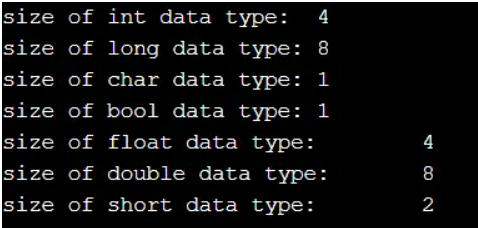Note: using sizeof() method we can identify the number of bytes each data type occupies in the main memory.

In the next article, I am going to discuss Variables in C++ with Examples. Here, in this article, I try to explain Primitive Data Types in C++ and I hope you enjoy this Primitive Data Types in C++ with Examples article.

### 2 thoughts on “Primitive Data Types in C++”

1.Shouldn’t the range for the integer be -“3276’7′ to 32767” instead of -“3276’8′ to 32767” in signed magnitude. -32768 is 1000 0000 0000 which literally means zero in signed state. -32767 will be represented as 1111 0000 0000. I am confused on why some people say it’s -32768 to 32767 and others websites also say it’s -32767 to 32767. What’s the real range in the c++14?

1.As an integer is 16-Bit or 2Byte, so it is going to store 65536 numbers. As it is signed, so it is going to store both positive and negative values. So, we need to divide 65536/2 i.e. 32,768. So, it is going to store 32,768 positive numbers as well as 32,768 negative numbers. The positive numbers will start from 0 up to 32,767 and the negative numbers will start from -1 up to -32,768. So, the minimum value integer data type can hold is -32,768 and the maximum value this data type can hold is 32,767. And if the size is 4 Bytes, then you can apply the same formula and calculate the result.